# Static Compiler Optimization Techniques We already examined the

• Slides: 10Static Compiler Optimization Techniques • We already examined the following static compiler techniques aimed at improving pipelined CPU performance: – Static pipeline scheduling (in ch 4. 1). – Loop unrolling (ch 4. 1). – Static branch prediction (in ch 4. 2). – Static multiple instruction issue: VLIW (in ch 4. 3). – Conditional or predicted instructions (in ch 4. 5) • Here we examine two additional static compiler-based techniques (in ch 4. 4): – Loop-Level Parallelism (LLP) analysis: • Detecting and enhancing loop iteration parallelism – GCD test. – Software pipelining (Symbolic loop unrolling). (In Chapter 4. 4) EECC 551 - Shaaban # Winter 2002 lec#7 1 -13 -2003Loop-Level Parallelism (LLP) Analysis • Loop-Level Parallelism (LLP) analysis focuses on whether data accesses in later iterations of a loop are data dependent on data values produced in earlier iterations. e. g. in for (i=1; i<=1000; i++) x[i] = x[i] + s; the computation in each iteration is independent of the previous iterations and the loop is thus parallel. The use of X[i] twice is within a single iteration. ÞThus loop iterations are parallel (or independent from each other). • Loop-carried Dependence: A data dependence between different loop iterations (data produced in earlier iteration used in a later one). • LLP analysis is normally done at the source code level or close to it since assembly language and target machine code generation introduces a loop-carried name dependence in the registers used for addressing and incrementing. • Instruction level parallelism (ILP) analysis, on the other hand, is usually done when instructions are generated by the compiler. EECC 551 - Shaaban # Winter 2002 lec#7 1 -13 -2003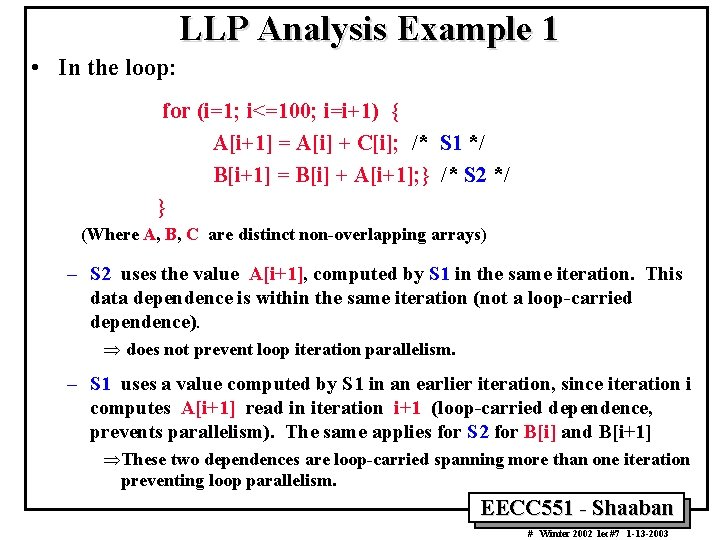LLP Analysis Example 1 • In the loop: for (i=1; i<=100; i=i+1) { A[i+1] = A[i] + C[i]; /* S 1 */ B[i+1] = B[i] + A[i+1]; } /* S 2 */ } (Where A, B, C are distinct non-overlapping arrays) – S 2 uses the value A[i+1], computed by S 1 in the same iteration. This data dependence is within the same iteration (not a loop-carried dependence). Þ does not prevent loop iteration parallelism. – S 1 uses a value computed by S 1 in an earlier iteration, since iteration i computes A[i+1] read in iteration i+1 (loop-carried dependence, prevents parallelism). The same applies for S 2 for B[i] and B[i+1] ÞThese two dependences are loop-carried spanning more than one iteration preventing loop parallelism. EECC 551 - Shaaban # Winter 2002 lec#7 1 -13 -2003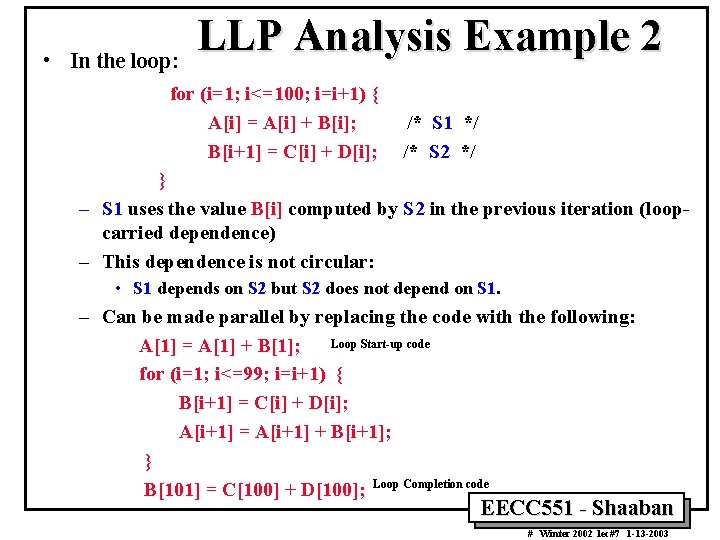• In the loop: LLP Analysis Example 2 for (i=1; i<=100; i=i+1) { A[i] = A[i] + B[i]; B[i+1] = C[i] + D[i]; /* S 1 */ /* S 2 */ } – S 1 uses the value B[i] computed by S 2 in the previous iteration (loopcarried dependence) – This dependence is not circular: • S 1 depends on S 2 but S 2 does not depend on S 1. – Can be made parallel by replacing the code with the following: A = A + B; Loop Start-up code for (i=1; i<=99; i=i+1) { B[i+1] = C[i] + D[i]; A[i+1] = A[i+1] + B[i+1]; } B = C + D; Loop Completion code EECC 551 - Shaaban # Winter 2002 lec#7 1 -13 -2003LLP Analysis Example 2 Original Loop: Iteration 1 for (i=1; i<=100; i=i+1) { A[i] = A[i] + B[i]; B[i+1] = C[i] + D[i]; } Iteration 2 A = A + B; A = A + B; B = C + D; B = C + D; . . . Loop-carried Dependence Iteration 99 Iteration 100 A = A + B; A = A + B; B = C + D; B = C + D; A = A + B; for (i=1; i<=99; i=i+1) { B[i+1] = C[i] + D[i]; A[i+1] = A[i+1] + B[i+1]; } B = C + D; Modified Parallel Loop: Loop Start-up code /* S 1 */ /* S 2 */ Iteration 1 A = A + B; A = A + B; B = C + D; B = C + D; . . Not Loop Carried Dependence Iteration 98 Iteration 99 A = A + B; A = A + B; B = C + D; B = C + D; Loop Completion code EECC 551 - Shaaban # Winter 2002 lec#7 1 -13 -2003ILP Compiler Support: Loop-Carried Dependence Detection • Compilers can increase the utilization of ILP by better detection of instruction dependencies. • To detect loop-carried dependence in a loop, the GCD test can be used by the compiler, which is based on the following: • If an array element with index: a x i + b is stored and element: c x i + d of the same array is loaded where index runs from m to n, a dependence exist if the following two conditions hold: 1 There are two iteration indices, j and k , m £ j , K £ n (within iteration limits) 2 The loop stores into an array element indexed by: a x j +b and later loads from the same array the element indexed by: c x k + d Thus: a x j + b = c x k + d EECC 551 - Shaaban # Winter 2002 lec#7 1 -13 -2003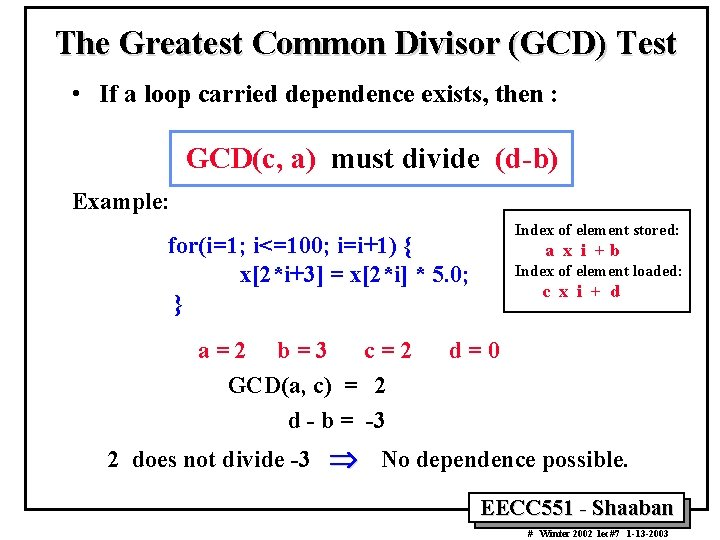The Greatest Common Divisor (GCD) Test • If a loop carried dependence exists, then : GCD(c, a) must divide (d-b) Example: Index of element stored: for(i=1; i<=100; i=i+1) { x[2*i+3] = x[2*i] * 5. 0; } a=2 b=3 c=2 GCD(a, c) = 2 d - b = -3 a x i +b Index of element loaded: c x i + d d=0 2 does not divide -3 Þ No dependence possible. EECC 551 - Shaaban # Winter 2002 lec#7 1 -13 -2003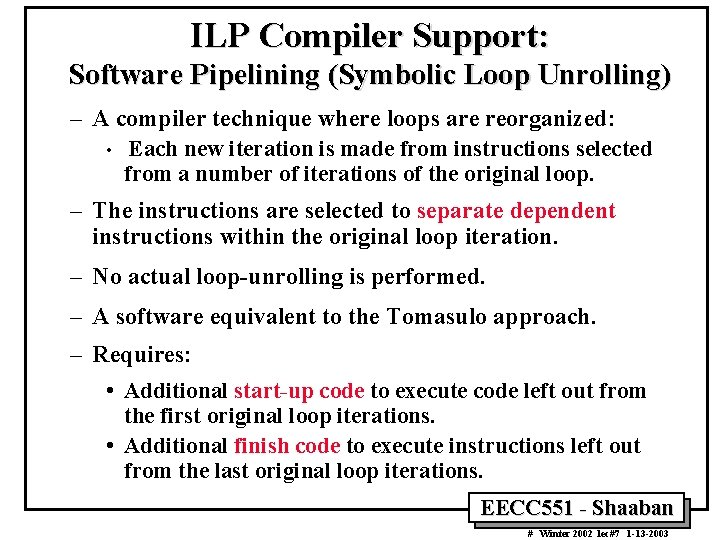ILP Compiler Support: Software Pipelining (Symbolic Loop Unrolling) – A compiler technique where loops are reorganized: • Each new iteration is made from instructions selected from a number of iterations of the original loop. – The instructions are selected to separate dependent instructions within the original loop iteration. – No actual loop-unrolling is performed. – A software equivalent to the Tomasulo approach. – Requires: • Additional start-up code to execute code left out from the first original loop iterations. • Additional finish code to execute instructions left out from the last original loop iterations. EECC 551 - Shaaban # Winter 2002 lec#7 1 -13 -2003Software Pipelining: Symbolic Loop Unrolling EECC 551 - Shaaban # Winter 2002 lec#7 1 -13 -2003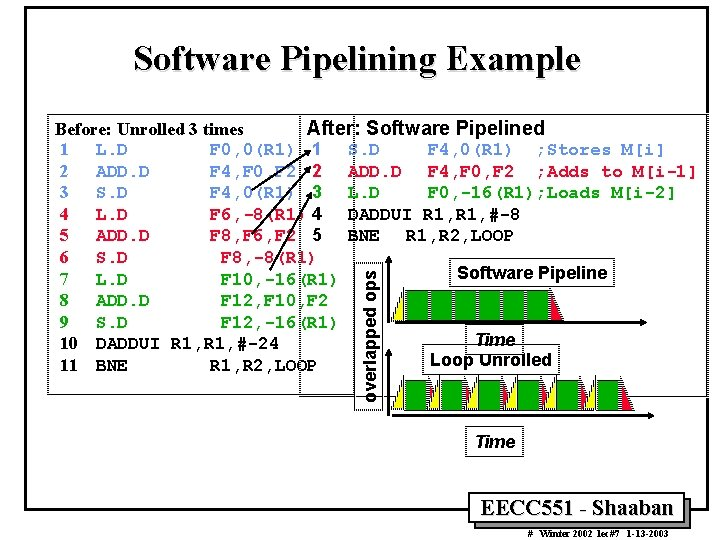Software Pipelining Example overlapped ops Before: Unrolled 3 times After: Software Pipelined 1 L. D F 0, 0(R 1) 1 S. D F 4, 0(R 1) ; Stores M[i] 2 ADD. D F 4, F 0, F 2 ; Adds to M[i-1] 3 S. D F 4, 0(R 1) 3 L. D F 0, -16(R 1); Loads M[i-2] 4 L. D F 6, -8(R 1) 4 DADDUI R 1, #-8 5 ADD. D F 8, F 6, F 2 5 BNE R 1, R 2, LOOP 6 S. D F 8, -8(R 1) Software Pipeline 7 L. D F 10, -16(R 1) 8 ADD. D F 12, F 10, F 2 9 S. D F 12, -16(R 1) Time 10 DADDUI R 1, #-24 Loop Unrolled 11 BNE R 1, R 2, LOOP Time EECC 551 - Shaaban # Winter 2002 lec#7 1 -13 -2003